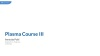# Learning Center

## Using the EEDF and Boltzmann Equation, Two-Term Approximation

In Part 5 of this course on plasma modeling using COMSOL Multiphysics® and the Plasma Module, we begin with an introduction to the electron energy distribution function (EEDF). You will learn what the function is and what the different options are for describing the distribution function in the Plasma interface. You will also see a comparison of each type of EEDF and gain an understanding of why and how their values differ, and what the EEDF means for your plasma model.

Furthermore, we will discuss how the EEDF affects the ionization rate constant depending on the function you use, how the electron transport properties have a weak dependence on the type of EEDF, and why it is usually best to start off with a Maxwellian EEDF.

Following these topics, we give an introduction to the Boltzmann Equation, Two-Term Approximation interface, such as what the interface computes and how it serves as a preprocessing step before the (space-dependent) plasma model is solved. We then outline the simple procedure for using this interface and discuss the additional settings that are necessary if the two-term approximation for the Boltzmann equation is used. These settings involve:

• Electron–electron collisions
• Secondary electron energy sharing
• Oscillating field
• Gas temperature
• If the electron–electron collisions property is active:
• Electron density
• Ionization degree
• Mole fractions

Following this, we provide an overview of the Mean Energies study and the Reduced Electric Fields study. You will then see a guided modeling demonstration where we perform an argon Boltzmann analysis, investigate an argon plasma chemistry, and compute the EEDF in different ways. The demonstration also shows how you can obtain cross-section data and files online for use in your plasma model. Lastly, we discuss space-dependent EEDF modeling and how an EEDF Initialization study is needed to provide initial conditions.